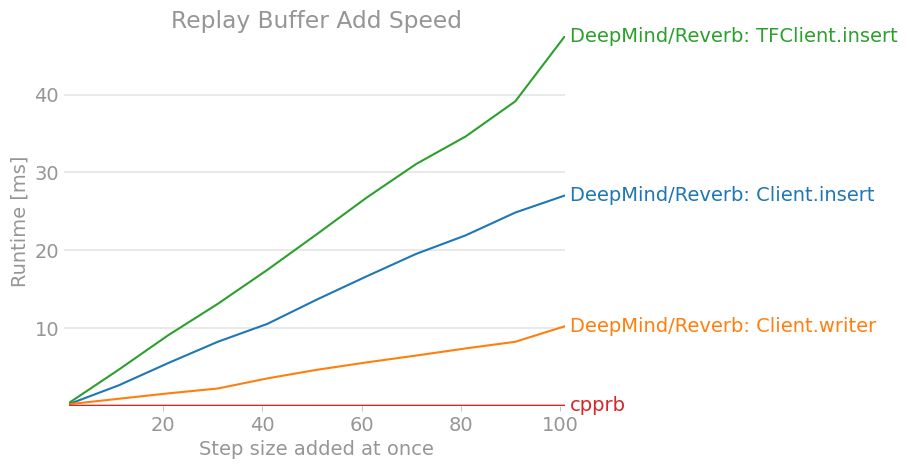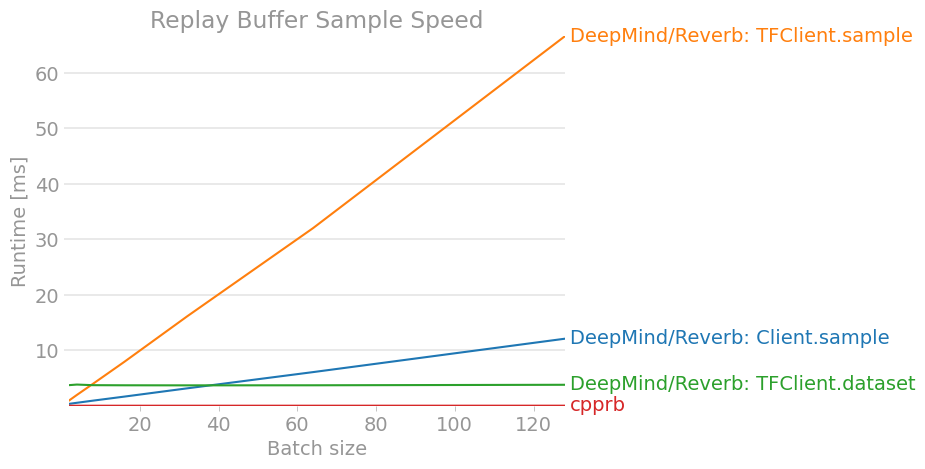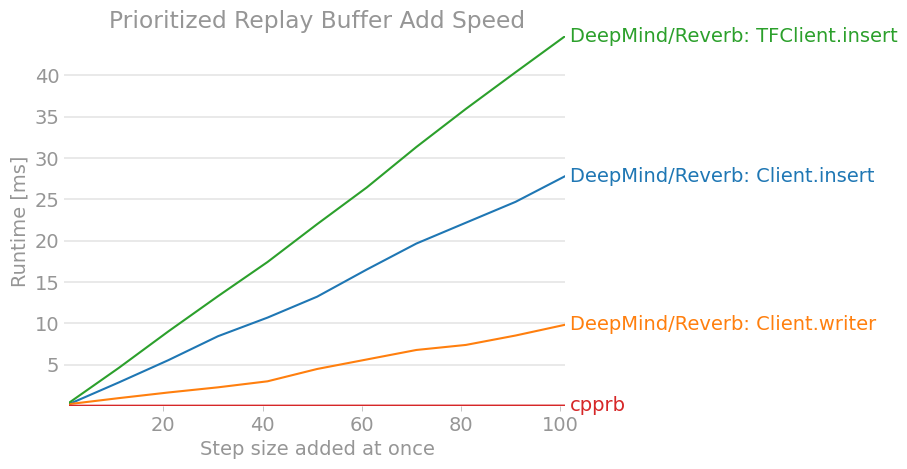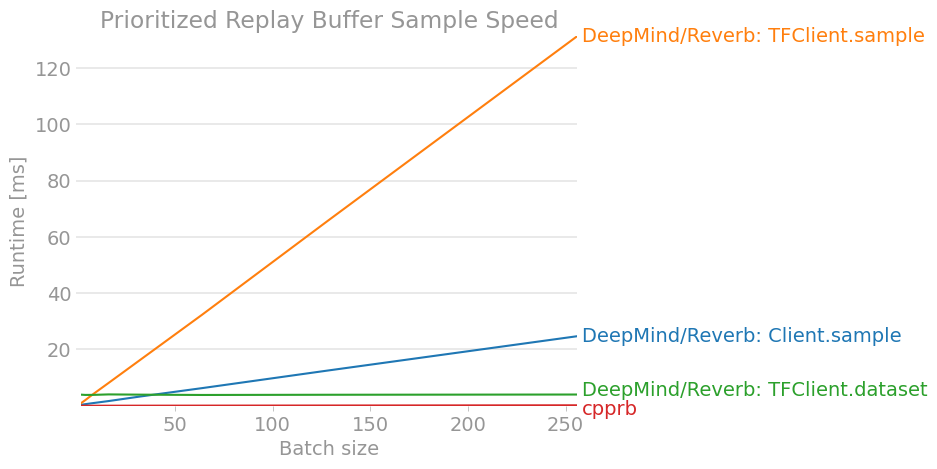# [PYTHON] [Reinforcement learning] DeepMind Experience Replay library Reverb usage survey [Client edition]

Japanese reprint of blog article posted in English

# 1.First of all

Continuing from Last time, about Reberb of DeepMind's Experience Replay library. This time, while reading the source code, I investigated the part not written in the README on the client side that instructs the data input / output operation.

# 2. `Client` and` TFClient`

Reverb uses a server-client model, but there are two client-side classes, `reverb.Client` and` reverb.TFClient`.

It is said that `Client` is for the early stages of development, and` TFClient` is used in the actual learning program. The big difference is that `TFClient`, as the name implies, is intended to be used in the computational graphs of TensorFlow.

One of the motivations for writing this organized article was that the APIs and usage of both were inconsistent and complicated.

The following article assumes that the server and client programs have been initialized as follows:

``````import numpy as np
import tensorflow as tf
import reverb

table_name = "ReplayBuffer"
alpha = 0.8
buffer_size = 1000
batch_size = 32

server = reverb.Server(tables=[reverb.Table(name=table_name,
sampler=reverb.selectors.Prioritized(alpha),
remover=reverb.selectors.Fifo(),
max_size=buffer_size,
rate_limiter=reverb.rate_limiters.MinSize(1))])

client = reverb.Client(f"localhost:{server.port}")
tf_client = reverb.TFClient(f"localhost:{server.port}")

obs = np.zeros((4,))
act = np.ones((1,))
rew = np.ones((1,))
next_obs = np.zeros_like(obs)
done = np.zeros((1,))
priority = 1.0

dtypes = [tf.float64,tf.float64,tf.float64,tf.float64,tf.float64]
shapes = [4,1,1,4,1]
``````

# 3. Preservation of transition or trajectory

3.1 `Client.insert`

``````client.insert([obs,act,rew,next_obs,done],priorities={table_name: priority})
``````

The `priorities` argument is` dict` because the same data can be registered in multiple tables (replay buffers) at the same time with different priorities. (I didn't know there was such a need)

You need to specify priority, even for regular non-Prioritized replay buffers.

`Client.insert` sends data to the server each time it is called

3.2 `Client.writer`

``````with client.writer(max_sequence_length=3) as writer:
writer.append([obs,act,rew,next_obs,done])
writer.create_item(table_name,num_timesteps=1,priority=priority)

writer.append([obs,act,rew,next_obs,done])
writer.append([obs,act,rew,next_obs,done])
writer.append([obs,act,rew,next_obs,done])
writer.create_item(table_name,num_timesteps=3,priority=priority) #Register 3 steps as 1 item.
#Send to server when exiting with block
``````

By using `reverb.Writer` returned by the` Client.writer` method as a context manager, You can set the contents to be saved more flexibly.

For example, the first half of the sample code above saves the same content as 3.1, but the second half saves the three steps together as one item. In other words, when sampling, you can sample all three as one. For example, it can be assumed that it is used when you want to sample each episode.

Data is sent to the server when `Writer.flush ()` or `Writer.close ()` is called (it is also called automatically when exiting the` with` block).

3.3 `TFClient.insert`

``````tf_client.inser([tf.constant(obs),
tf.constant(act),
tf.constant(rew),
tf.constant(next_obs),
tf.constant(done)],
tablea=tf.constant([table_name]),
priorities=tf.constant([priority],dtype=tf.float64))
``````

The `tables` argument is a rank 1` tf.Tensor` of `str`, and the` priorities` is a rank 1 `tf.Tensor` of` float64` (which would otherwise be a `float32`). Both `shape`s must match.

It is probably necessary to keep the `tf.Tensor` of each data at rank 1 or higher for later sampling.

## 3.4 Summary

Send to server Multiple steps into one item Use in TF calculation graph data
`Client.insert` Every time X X anything is fine
`Client.writer` `Writer.close()`, `Writer.flush()` (Including`with`When leaving) O X anything is fine
`TFClient.insert` Every time X O `tf.Tensor`

# 4. Reading a transition or trajectory

Neither method corresponds to the β parameter of weight correction in Prioritized Experience Replay. (It doesn't calculate the weight of priority sampling in the first place)

4.1 `Client.sample`

``````client.sample(table_name,num_samples=batch_size)
``````

The return value is the `generator` of` reverb.replay_sample.ReplaySample`. `ReplaySample` is a named Tuple and has ʻinfo` and` data`. `Data` contains the saved data, and ʻinfo` contains information such as key and priority.

4.2 `TFClient.sample`

``````tf_client.sample(tf.constant([table_name]),data_dtypes=dtypes)
``````

Unfortunately, this method does not support batch sampling. The return value will be `ReplaySample`.

4.3 `TFClient.dataset`

``````tf_client.dataset(tf.constant([table_name]),dtypes=dtypes,shapes=shapes)
``````

It adopts a method that is completely different from other methods, and it seems that it is mainly intended to adopt this method in large-scale production learning.

The return value of this function is `reverb.ReplayDataset`, which inherits` tf.data.Dataset`. This `ReplayDataset` can pull out` ReplaySample` like `generator`, and it will automatically fetch data from the server at the right time. In other words, instead of `sample` every time, once you set` ReplayDataset`, it will continue to eject the automatically saved data.

Since `shapes` raises an error when` 0` is specified as an element, it seems necessary to save the data at rank 1 or higher.

Other parameters for performance adjustment are detailed to write here, so check the Comment in the source code. I want you to.

## 4.4 Summary

Batch output Return type Type specification Designation of shape
`Client.sample` O `replay_sample.ReplaySample`of`generator` Unnecessary Unnecessary
`TFClient.sample` X `replay_sample.ReplaySample` necessary Unnecessary
`TFClient.dataset` O (Automatically implemented internally) `ReplayDataset` necessary necessary

# 5. Update of priority

Unlike other replay buffer implementations, the ID that specifies the element is not a serial number starting with `0`, but a seemingly random hash. It can be accessed with `ReplaySample.info.key`. (Since it is difficult to write, I will omit some of the sample code. I'm sorry.)

5.1 `Client.mutate_priorities`

``````client.mutate_priorities(table_name,updates={key:new_priority},deletes=[...])
``````

This can be deleted as well as updated.

5.2 `TFClient.update_priorities`

``````tf_client.update_priorities(tf.constant([table_name]),
keys=tf.constant([...]),
priorities=tf.constant([...],dtype=tf.float64))
``````

# 6. Performance comparison

Since it's a big deal, I also benchmarked my work cpprb.

** Note: Reinforcement learning is not determined solely by the speed of the replay buffer, as there are other heavy processes such as deep learning learning and environment updates. (On the other hand, depending on the implementation and conditions of the replay buffer, it seems that the processing time of the replay buffer and the processing time of deep learning may be about the same.) **

Benchmark was executed in the environment by the following Dockerfile.

#### `Dockerfile`

``````
FROM python:3.7

RUN apt update \
&& apt install -y --no-install-recommends libopenmpi-dev zlib1g-dev \
&& apt clean \
&& rm -rf /var/lib/apt/lists/* \
&& pip install tf-nightly==2.3.0.dev20200604 dm-reverb-nightly perfplot

# Reverb requires development version TensorFlow

CMD ["bash"]
``````

(Since it is implemented on the CI of the repository of cpprb, cpprb is also installed additionally. It is almost synonymous with `pip install cpprb`)

Then, I ran the following benchmark script and drew a graph of execution time.

#### `benchmark.py`

``````
import gc
import itertools

import numpy as np
import perfplot
import tensorflow as tf

# DeepMind/Reverb: https://github.com/deepmind/reverb
import reverb

from cpprb import (ReplayBuffer as RB,
PrioritizedReplayBuffer as PRB)

# Configulation
buffer_size = 2**12

obs_shape = 15
act_shape = 3

alpha = 0.4
beta  = 0.4

env_dict = {"obs": {"shape": obs_shape},
"act": {"shape": act_shape},
"next_obs": {"shape": obs_shape},
"rew": {},
"done": {}}

# Initialize Replay Buffer
rb  =  RB(buffer_size,env_dict)

# Initialize Prioritized Replay Buffer
prb  =  PRB(buffer_size,env_dict,alpha=alpha)

# Initalize Reverb Server
server = reverb.Server(tables =[
reverb.Table(name='ReplayBuffer',
sampler=reverb.selectors.Uniform(),
remover=reverb.selectors.Fifo(),
max_size=buffer_size,
rate_limiter=reverb.rate_limiters.MinSize(1)),
reverb.Table(name='PrioritizedReplayBuffer',
sampler=reverb.selectors.Prioritized(alpha),
remover=reverb.selectors.Fifo(),
max_size=buffer_size,
rate_limiter=reverb.rate_limiters.MinSize(1))
])

client = reverb.Client(f"localhost:{server.port}")
tf_client = reverb.TFClient(f"localhost:{server.port}")

# Helper Function
def env(n):
e = {"obs": np.ones((n,obs_shape)),
"act": np.zeros((n,act_shape)),
"next_obs": np.ones((n,obs_shape)),
"rew": np.zeros(n),
"done": np.zeros(n)}
return e

""" Add for Reverb Client
"""
n = e["obs"].shape
with _rb.writer(max_sequence_length=1) as _w:
for i in range(n):
_w.append([e["obs"][i],
e["act"][i],
e["rew"][i],
e["next_obs"][i],
e["done"][i]])
_w.create_item(table,1,1.0)

""" Add for Reverb Client
"""
n = e["obs"].shape
for i in range(n):
_rb.insert([e["obs"][i],
e["act"][i],
e["rew"][i],
e["next_obs"][i],
e["done"][i]],priorities={table: 1.0})

""" Add for Reverb TFClient
"""
n = e["obs"].shape
for i in range(n):
_rb.insert([tf.constant(e["obs"][i]),
tf.constant(e["act"][i]),
tf.constant(e["rew"][i]),
tf.constant(e["next_obs"][i]),
tf.constant(e["done"])],
tf.constant([table]),
tf.constant([1.0],dtype=tf.float64))

def sample_client(_rb,table):
""" Sample from Reverb Client
"""
def sample(n):
return [i for i in _rb.sample(table,num_samples=n)]

return sample

def sample_tf_client(_rb,table):
""" Sample from Reverb TFClient
"""
def sample(n):
return [_rb.sample(table,
[tf.float64,tf.float64,tf.float64,tf.float64,tf.float64])
for _ in range(n)]

return sample

def sample_tf_client_dataset(_rb,table):
""" Sample from Reverb TFClient using dataset
"""
def sample(n):
dataset=_rb.dataset(table,
[tf.float64,tf.float64,tf.float64,tf.float64,tf.float64],
[4,1,1,4,1])
return itertools.islice(dataset,n)
return sample

setup = env,
time_unit="ms",
labels = ["DeepMind/Reverb: Client.insert",
"DeepMind/Reverb: Client.writer",
"DeepMind/Reverb: TFClient.insert",
"cpprb"],
n_range = [n for n in range(1,102,10)],
xlabel = "Step size added at once",
title = "Replay Buffer Add Speed",
logx = False,
logy = False,
equality_check = None)

# Fill Buffers
for _ in range(buffer_size):
o = np.random.rand(obs_shape) # [0,1)
a = np.random.rand(act_shape)
r = np.random.rand(1)
d = np.random.randint(2) # [0,2) == 0 or 1
client.insert([o,a,r,o,d],priorities={"ReplayBuffer": 1.0})

# ReplayBuffer.sample
perfplot.save(filename="ReplayBuffer_sample2.png ",
setup = lambda n: n,
time_unit="ms",
kernels = [sample_client(client,"ReplayBuffer"),
sample_tf_client(tf_client,"ReplayBuffer"),
sample_tf_client_dataset(tf_client,"ReplayBuffer"),
rb.sample],
labels = ["DeepMind/Reverb: Client.sample",
"DeepMind/Reverb: TFClient.sample",
"DeepMind/Reverb: TFClient.dataset",
"cpprb"],
n_range = [2**n for n in range(1,8)],
xlabel = "Batch size",
title = "Replay Buffer Sample Speed",
logx = False,
logy = False,
equality_check=None)

time_unit="ms",
setup = env,
labels = ["DeepMind/Reverb: Client.insert",
"DeepMind/Reverb: Client.writer",
"DeepMind/Reverb: TFClient.insert",
"cpprb"],
n_range = [n for n in range(1,102,10)],
xlabel = "Step size added at once",
title = "Prioritized Replay Buffer Add Speed",
logx = False,
logy = False,
equality_check=None)

# Fill Buffers
for _ in range(buffer_size):
o = np.random.rand(obs_shape) # [0,1)
a = np.random.rand(act_shape)
r = np.random.rand(1)
d = np.random.randint(2) # [0,2) == 0 or 1
p = np.random.rand(1)

client.insert([o,a,r,o,d],priorities={"PrioritizedReplayBuffer": p})

perfplot.save(filename="PrioritizedReplayBuffer_sample2.png ",
time_unit="ms",
setup = lambda n: n,
kernels = [sample_client(client,"PrioritizedReplayBuffer"),
sample_tf_client(tf_client,"PrioritizedReplayBuffer"),
sample_tf_client_dataset(tf_client,"PrioritizedReplayBuffer"),
lambda n: prb.sample(n,beta=beta)],
labels = ["DeepMind/Reverb: Client.sample",
"DeepMind/Reverb: TFClient.sample",
"DeepMind/Reverb: TFClient.dataset",
"cpprb"],
n_range = [2**n for n in range(1,9)],
xlabel = "Batch size",
title = "Prioritized Replay Buffer Sample Speed",
logx=False,
logy=False,
equality_check=None)
``````

The result is as follows. (The results may be out of date, so you can check the latest version at cpprb project site.)# 7. Conclusion

We investigated and organized how to use the Reverb client of the DeepMind Experience Replay framework. Compared to other replay buffer implementations such as OpenAI / Baselines, I found it difficult to understand because there are many differences in API and usage. (I hope it will be a little easier to understand by the time the stable version is released.)

At the very least, it wouldn't seem to be superior in terms of performance unless large-scale distributed learning was performed and all reinforcement learning was completed within the TensorFlow calculation graph.

Of course, large-scale distributed learning and reinforcement learning in computational graphs have the potential to significantly improve performance, so I think we need to continue to consider them.